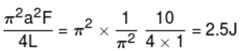Courses

# JEE Main Physics Mock - 4

## 25 Questions MCQ Test Mock Test Series for JEE Main & Advanced 2021 | JEE Main Physics Mock - 4

Description
This mock test of JEE Main Physics Mock - 4 for JEE helps you for every JEE entrance exam. This contains 25 Multiple Choice Questions for JEE JEE Main Physics Mock - 4 (mcq) to study with solutions a complete question bank. The solved questions answers in this JEE Main Physics Mock - 4 quiz give you a good mix of easy questions and tough questions. JEE students definitely take this JEE Main Physics Mock - 4 exercise for a better result in the exam. You can find other JEE Main Physics Mock - 4 extra questions, long questions & short questions for JEE on EduRev as well by searching above.
QUESTION: 1

Solution:
QUESTION: 2

Solution:
QUESTION: 3

### For a given material, the Young's modulus is 2.4 times that of rigidity modulus. Its Poisson's ratio is

Solution: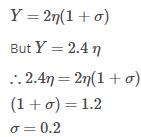QUESTION: 4

If 3.8 x 10-6 is added to 4.2 x 10-5 giving due regard to significant figures, then the result will be

Solution:
QUESTION: 5
Two spherical conductors of radii 4 cm and 5 cm are charged to the same potential. If σ₁ and σ₂ be the respective values of the surface density of charge on both the conductors, then the ratio σ₁/σ₂ is
Solution:
QUESTION: 6
A transformer is used to reduce the mains supply of 220 V to 11 V. If the currents in primary and secondary coils are 5 A and 90 A respectively, efficiency of transformer is
Solution: Efficiency= (output power/input power)*100
=>(90*11/220*5)*100
90%
QUESTION: 7

A parallel plate capacitor is first charged and then a dielectric slab is introduced between the plates after disconnecting it from the battery. The quantity that remains unchanged is

Solution:
QUESTION: 8
Spot the wrong statement : The acceleration due to gravity decreases if
Solution:
QUESTION: 9
For Boyle's law to hold the gas should be
Solution:
QUESTION: 10

When two deuterium nuclei fuse together, in addition to tritium, we get a

Solution:QUESTION: 11

If two bodies are projected at 30o and 60o respectively, with the same velocity, then

Solution:
QUESTION: 12
In the following question, a Statement of Assertion (A) is given followed by a corresponding Reason (R) just below it. Read the Statements carefully and mark the correct answer-
Assertion(A): A uniform solid cylinder rolling with angular velocity ω along a plane surface strikes a vertical rigid wall. Angular velocity of cylinder when it begins to roll up a wall is less than the initial Angular velocity (ω).
Reason(R): After striking the vertical wall angular velocity increases.
Solution:
QUESTION: 13
In the following question, a Statement of Assertion (A) is given followed by a corresponding Reason (R) just below it. Read the Statements carefully and mark the correct answer-
Assertion(A): Current is passed through a metallic wire heating it red. When cold water is poured on half of its portion, the rest of half portion becomes more hot.
Reason(R): Resistance decreases due to decrease in temperature and then current through wire increases.
Solution:
QUESTION: 14
A disc is placed on a surface of pond which has refractive index 5/3. A source of light is placed 4 m below the surface of liquid. The minimum radius of disc needed so that light is not coming out is
Solution:
QUESTION: 15
Sound waves transfer
Solution:
QUESTION: 16

The wavelength of ultrasonic waves in air is of the order of

Solution:
QUESTION: 17

70 calories of heat are required to raise the temperature of 2 moles of an ideal gas at constant pressure from 30oC to 35oC. The amount of heat required to raise the temperature of the same sample of the gas through the same range at constant volume is nearly (Gas constant = 1.99 cal/K-mole)

Solution:
Cp= q/n*delta t
= 70/2*5=7
We know that for diatomic gas
Cp/Cv=7/5
So Cv=5
Cv=q/n*delta t
5=q/2*5
q=50 calories
QUESTION: 18
Both light and sound waves produce diffraction. It is more difficult to observe the diffraction with light waves because
Solution:
QUESTION: 19

A boy of mass 42 Kg eats bananas of 980 calories. If this energy is used up to lift him from the ground, the height to which he can move up is

Solution:

Height = h.
Potential Energy (P.E) = 980 Calories = 4100.32 Jule
Now P.E = mgh
4100.32 = 42 x 9.8 x h
h = 4100.32/42x9.8 ≈ 10m

QUESTION: 20

In the given circuit the capacitor (C) may be charged through resistance R by battery V by closing switch S1. Also when S1 is opened and S2 is closed the capacitor is connected in series with inductor (L).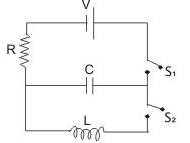Q. At the start, the capacitor was uncharged. When switch S1 is closed and S2 is kept open, the time constant of this circuit is τ. Which of the following is correct?

Solution:
*Answer can only contain numeric values
QUESTION: 21

A plastic circular disc of radius 10 cm is placed on a thin oil film of thickness 2 mm, spread over a flat horizontal surface. The torque (N-m) required to spin the disc about its central vertical axis with a constant angular velocity 8 rad/sec is  (coefficient of viscosity of oil is 1.0 kg/m-s) (take π = 3.14)

Solution: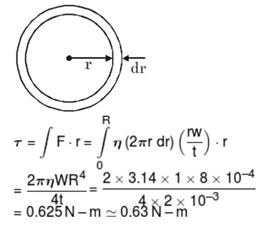*Answer can only contain numeric values
QUESTION: 22

Calculate the value of output voltage V0 (in V) if the Si diode and Ge diode conduct at 0.7 V and 0.3V respectively as shown in figure.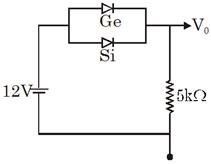Solution: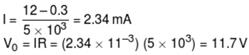*Answer can only contain numeric values
QUESTION: 23

In Young's double slit experiment, the wavelength of red light is 7800 Å and that of blue light is 5200 Å. The minimum value of n for which nth bright band due to red light coincides with (n + 1)th bright band due to blue light, is :

Solution:

n (7800) = (n + 1) 5200

*Answer can only contain numeric values
QUESTION: 24

A railway track (made of iron) is laid in winter when the average temperature is 18°C. The track consists of sections of 12.0 m  placed on after the other. How much gap (in cm) should be left between two such sections so that there is no compression during summer when the maximum temperature goes to 48°C ? Coefficient of linear expansion of iron = 11 × 10–6/°C.

Solution:

ΔL = L0 α ΔT
= 12 × 11 × 10–6 × 30
= 3960 × 10–6 m

*Answer can only contain numeric values
QUESTION: 25

A string of mass per unit length μ is clamped at both ends such that one end of the string is at x = 0 and the other end at x = L. When string vibrates in fundamental mode, amplitude of the midpoint of string is a and tension in string is F. Find the total oscillation energy (in J) stored in the string. (Use L = 1m, F = 10 N,  a = 1/πm)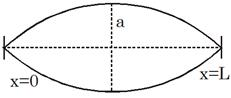Solution: